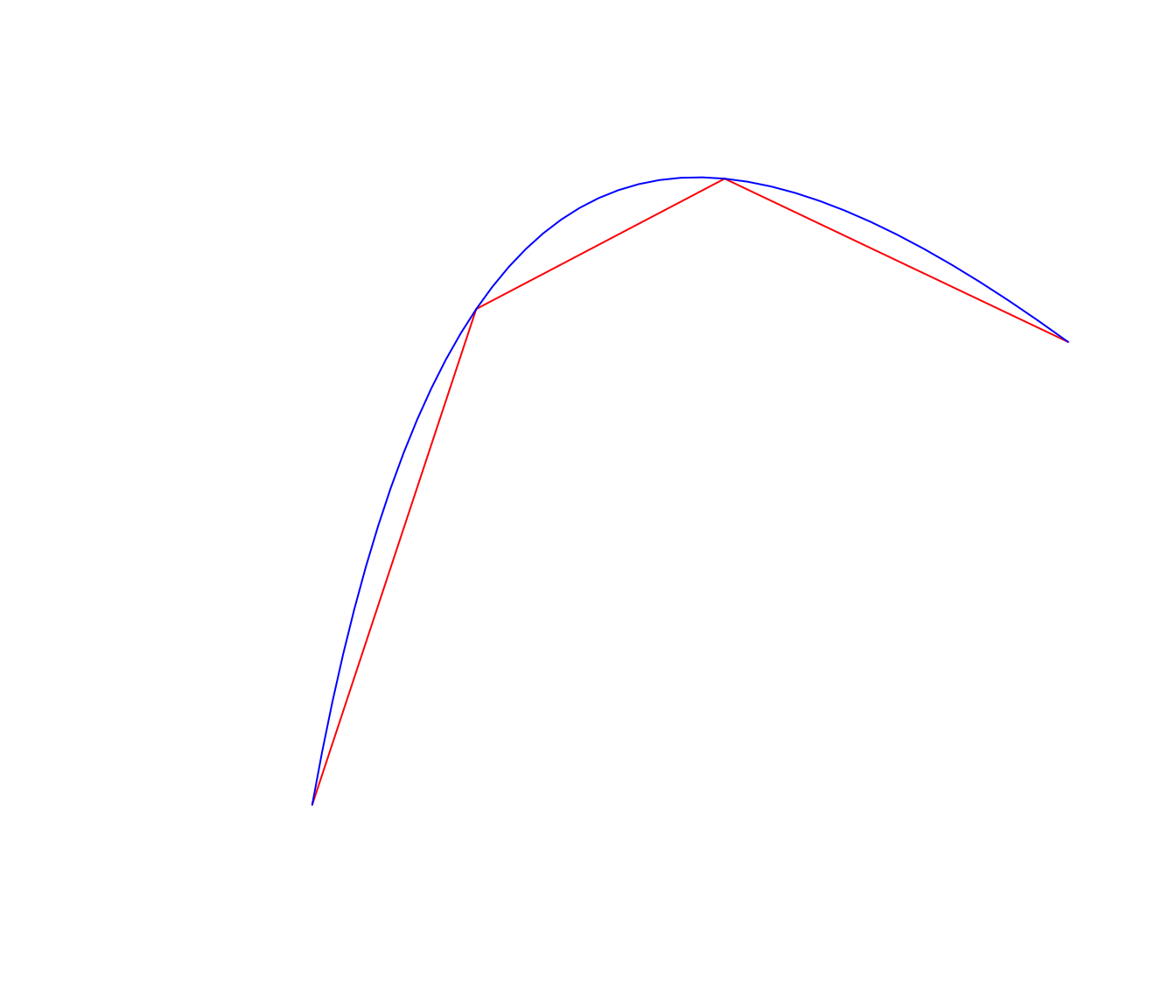# Fast(?) cubic beziers at variable resolution

• I wanted to calculate some points along cubic bezier curves
• Current R grid bezier implementation is not a real bezier! According to docs for grid::bezierGrob(): “The curve is currently drawn using an approximation based on X-splines.”
• Code below is the fastest thing I could come up with (I’m only 80% sure it’s correct)
• It vectorized implemntation of the explicit form of the curve as explained on Wikipedia
• I need something that can quickly change between low-complexity and high-complexity approximations to the curve by changing the number of line segments generated
• Speed-ups welcomed

## Code

#~~~~~~~~~~~~~~~~~~~~~~~~~~~~~~~~~~~~~~~~~~~~~~~~~~~~~~~~~~~~~~~~~~~~~~~~~~~~~
# Pre-calculate sets of control-point weights for each for a range of
# different desired points in the output
#~~~~~~~~~~~~~~~~~~~~~~~~~~~~~~~~~~~~~~~~~~~~~~~~~~~~~~~~~~~~~~~~~~~~~~~~~~~~~
bf3s <- lapply(1:100, function(N) {
t  <- seq.int(0, 1, length.out = N)

matrix(c(
1 * t^0 * (1 - t)^3,
3 * t^1 * (1 - t)^2,
3 * t^2 * (1 - t)^1,
1 * t^3 * (1 - t)^0
), ncol = 4
)
})

#~~~~~~~~~~~~~~~~~~~~~~~~~~~~~~~~~~~~~~~~~~~~~~~~~~~~~~~~~~~~~~~~~~~~~~~~~~~~~
#' Convert cubic  beziers to data.frame of coordinates along curve
#'
#' @param x,y coords of control points
#' @param N Number of output points
#'
#' @return list of coords
#~~~~~~~~~~~~~~~~~~~~~~~~~~~~~~~~~~~~~~~~~~~~~~~~~~~~~~~~~~~~~~~~~~~~~~~~~~~~~
bezier3 <- function(x, y, N = 5) {
stopifnot(length(x)== 4, length(y) == 4)
list(
x = rowSums(bf3s[[N]] * matrix(x, nrow = N, ncol = 4, byrow = TRUE)),
y = rowSums(bf3s[[N]] * matrix(y, nrow = N, ncol = 4, byrow = TRUE))
)
}

## Demo

library(grid)

# Random control points
set.seed(1)
xcp <- runif(4)
ycp <- runif(4)

# Generate low and high complexity approximations
pt_lo <- bezier3(xcp, ycp,  4)
pt_hi <- bezier3(xcp, ycp, 40)

# Draw my approximations
grid.lines(pt_lo$x, pt_lo$y, gp = gpar(col = 'red' ))
grid.lines(pt_hi$x, pt_hi$y, gp = gpar(col = 'blue'))## Comparison to grid::grid.bezier()

# Overlay the grid version of this bezier
grid.lines(pt_lo$x, pt_lo$y, gp = gpar(col = 'red' ))
grid.lines(pt_hi$x, pt_hi$y, gp = gpar(col = 'blue'))
grid.bezier(xcp, ycp)